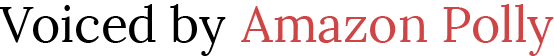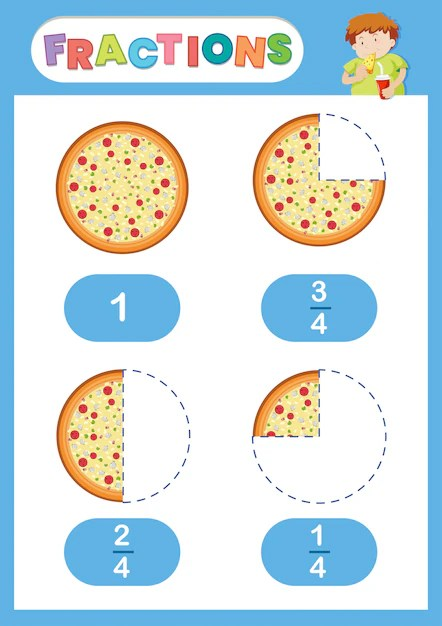# The Importance of Understanding Fractions: Tips and Strategies for 7th graders

 Would You Rather Listen to the Lesson?Understanding fractions is a crucial aspect of mathematical literacy. As a 7th grader, it is important to have a solid grasp of fractions as they form the building blocks of many advanced mathematical concepts, such as decimals, ratios, and percentages. In this article, we will discuss the importance of understanding fractions, along with some tips and strategies to help 7th graders improve their understanding of this topic.

### Fractions as a way to represent parts of a whole

First and foremost, it is important to understand that fractions are a way to represent parts of a whole. They allow us to express quantities that cannot be represented by whole numbers, and are used in many real-world applications, such as cooking recipes, measuring lengths, and calculating distances. Understanding fractions is, therefore, a fundamental part of mathematical literacy and is essential for success in both academic and professional life.

A fraction can be demonstrated as a part of a whole through several methods, including:

1. Fraction strips or circles: This involves dividing a strip or circle into equal parts and labeling each part with the corresponding fraction. This can help show the relationship between the fraction and the whole.
2. Visual representation: Dividing an object, such as a pizza, into parts and labeling each part with the corresponding fraction can help illustrate the concept of a fraction as a part of a whole.
3. Area models: Creating an area model of a fraction, such as shading a portion of a rectangle to represent the fraction, can help demonstrate how the fraction represents a part of the whole.
4. Number line representation: Representing a fraction on a number line can help demonstrate how the fraction relates to the whole. For example, if the whole is represented by 1, a fraction such as 1/2 would be represented by a point halfway along the number line.

Using these methods, along with real-world examples, can help make the abstract concept of fractions more concrete and tangible, making it easier to understand and demonstrate the relationship between a fraction and the whole.Tips and strategies for understanding fractions begin with demonstrating that a fraction is a piece of a larger whole

### Differentiating the numerator and the denominator

To improve your students’ understanding of fractions, it is important to start with the basics. Understanding the numerator and denominator and how they work together to represent a fractional part of a whole is key. Additionally, 7th graders should be familiar with the basic operations of fractions, such as addition, subtraction, multiplication, and division. Practicing these operations with different types of fractions, such as proper, improper, and mixed numbers, will help build a strong foundation in this topic.

To teach a student to differentiate a numerator from a denominator, you can use the following steps:

1. Introduce the concept of fractions: Here, we circle back to our first tip… Begin by explaining that a fraction is a way to represent a part of a whole. Use real-world examples, such as a pizza divided into slices, to help illustrate the concept.
2. Define the numerator and denominator: Explain that the fraction is made up of two parts: the numerator and the denominator. The numerator represents the number of parts being considered, while the denominator represents the total number of parts in the whole.
3. Use visual aids: Use diagrams, such as fraction strips or circles, to help demonstrate the relationship between the numerator and denominator. This can help students better understand the concept of a fraction and how the numerator and denominator work together.
4. Practice identifying the numerator and denominator: Provide students with several examples of fractions and have them identify the numerator and denominator for each one. Emphasize the importance of understanding which number represents the numerator and which represents the denominator.
5. Reinforce the concept: Regularly review the concept of the numerator and denominator in different contexts. This can help students solidify their understanding and avoid confusion.

By using these steps, along with patience and clear explanations, you can help students differentiate the numerator from the denominator and build a strong foundation in the concept of fractions.

### Visualising to help understand fractions

Another helpful strategy for improving your understanding of fractions is to visualize them. Draw diagrams or models to help you understand the concept of dividing a whole into parts. For example, you can use fraction strips or circles to demonstrate how the numerator represents a portion of the denominator. This can be particularly helpful for understanding equivalent fractions, which have the same value even though their numerators and denominators may be different.

There are several ways to visualize fractions, including:

1. Fraction strips: A fraction strip is a rectangular strip that is divided into equal parts, each representing a fraction. This can help students understand the relationship between the fraction and the whole.
2. Circles or pies: A circle or pie can be divided into parts to represent fractions. This can help students understand that a fraction is a part of a whole.
3. Area models: An area model involves shading a portion of a rectangle to represent a fraction. This can help students understand how the fraction relates to the whole and how it can be compared to other fractions.
4. Number lines: Representing fractions on a number line can help students understand the relationship between the fraction and the whole. For example, if the whole is represented by 1, a fraction such as 1/2 would be represented by a point halfway along the number line.
5. Real-world examples: Using real-world examples, such as dividing a pizza into slices or sharing candy among friends, can help students understand the concept of a fraction and how it relates to the whole.

Using these methods, along with clear explanations and opportunities for hands-on practice, can help students understand and visualize the concept of fractions.

### Real-world practice (makes perfect)

It is also important to practice with real-world examples to make the concept of fractions more meaningful. For example, try using fractions to calculate the amount of ingredients needed for a recipe or to divide a length into equal parts. This can help make the abstract concept of fractions more concrete and tangible.

Practicing real-world examples of fractions can help students understand the concept and see its relevance in everyday life. Here are some ways to practice real-world examples of fractions:

1. Cooking and baking: Measuring ingredients using fractions, such as half a cup of sugar or a quarter of a teaspoon of salt, can help students understand the concept of a fraction in practical terms.
2. Money: Using coins and dollars to demonstrate fractions of a dollar, such as 25 cents for a quarter, can help students understand the concept of a fraction and its real-world application.
3. Sharing objects: Dividing objects, such as candy or toys, into equal parts and assigning each part a fraction can help students understand the relationship between the fraction and the whole.
4. Time: Practicing telling time using fractions, such as 15 minutes past the hour or half past the hour, can help students understand the concept of a fraction and its application to time.
5. Measurement: Using fractions to measure length, weight, or volume can help students understand the concept of a fraction and its real-world application.

By incorporating real-world examples into the practice of fractions, students can better understand the concept and see its relevance in everyday life, which can help make the learning experience more engaging and meaningful.

### Help is at hand

Getting fractions right is a really important step in a 7th grader’s math education. This is why it’s so important that you use creativity to engage your students and help them make meaningful connections between this abstract concept and the real-world applications of it.

So, don’t be afraid to ask for help or seek additional resources. There are thousands of online resources out there, but why waste time trawling through them because they’re not good enough, lack sufficient educational value, or are incomplete…

Instead, save yourself hours of time and lessen the frustration by becoming a member of MathTeacherCoach! We’ve built curriculums from 3rd grade all the way through to Pre-Calculus for the entire year, so you’ll never have to plan a lesson again! Simply download your chosen lesson, shave hours off of grading (because we’ve provided the work!), and when you close the classroom door each day, you get spend your time where you WANT TO.